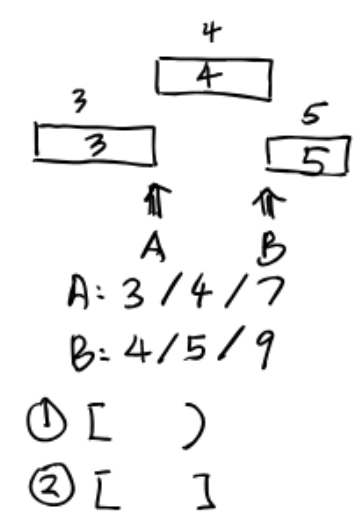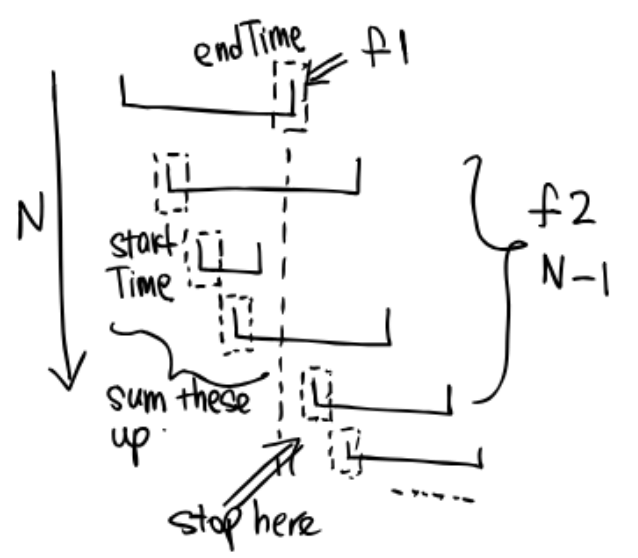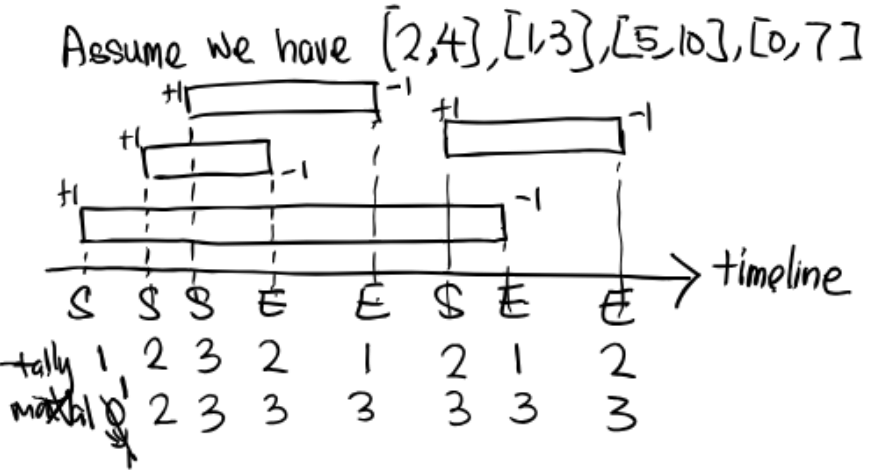My HeapPQ Implementation (Based on Approach 3B): link

It allows comparators to support MinPQ and MaxPQ. It includes testing.

Video solution by a 61B TA: link (It’s great!)

## Flight Problem

### Start-End Overlap

Consider the overlap situation:According to the comment in FlightSolver.java:

If a flight starts at the same time as a flight’s end time, they are considered to be in the air at the same time.

So the lab goes for the 2nd option. That is, at the time of A, the number of passengers should be 7.

I have commented the difference in the code. Just a tiny modification on a relational operator.

### Brute Force• Sort the array of flights by startTime of each flight. => $O(N\log{N})$
• Iterate the array, and compare the current flight f1 with the flight f2 of the rest of the array. => Worst case: $\Theta(N^2)$
• If the startTime of f2 is less (or equal for Start-End Overlap Option 1) than the endTime of f1, consider these two flights overlap.
• Iterate the rest of the array to sum up all overlapping flights.
• Stop when reach the end of the array or the startTime of f2 is larger (or equal, same as above) than the endTime of f1.
• Compare the sum value with the recorded max value, and update if necessary.

So the total runtime is $O(N^2)$.

### Simplify The Problem

Consider setting all passenger numbers to 1:The problem becomes simpler: Return the maximum number of flights in the air at the same time, that is, how many blocks overlap for a given time.

We can map the startTime and endTime on the timeline as shown in the graph. There are $2N$ of entries and exits on the timeline. Each time we enter a block, plus 1; each time we exit a block, minus 1.

A variable tally records the the number of overlapping blocks as time goes by. So we need another variable maxVal to record the possible maximum value.

Implementation:

We can map the Entry array and the Exit array into an $2N$ array with both entries and exits.

Since we need to know whether the item is an Entry or Exit, it is a bit complicated to implement the algorithm (but it’s indeed intuitive and the runtime is guaranteed theoretically).

And finally go through the array and record the values of tally and maxVal.

Runtime:

• Sort the Entry array => $O(N\log{N})$
• Sort the Exit array => $O(N\log{N})$
• Mergesort => $O(N\log{N})$
• Go through and record => $O(2N) \sim O(N)$

So the total runtime is $O(N\log{N})$.

In the flight problem, we just need to change the values of increment and decrement.

### Using Two HeapPQs

A better approach to tackle this problem is to use two HeapPQs, one for startTime and the other for endTime. (It’s more intuitive if you refer to the graph above)

Go through the flight array and add the flight into the two heaps.

Set up necessary values to record tally and the max value. While the heaps are not empty, we need to “pop” the values from them and compare.

If the startTime from minStartPQ is less than or equal (“before”) the endTime from minEndPQ, we increase the tally; on the other hand, we decrease the tally.

Finally, after each round update the maxVal if necessary.

Runtime:

Assume we have a flight array in random, so adding all flights into both heaps require:

$$O(\log{1} + \log{2} + \ldots + \log{N}) = O(\log{N!})\leq O(\log{N} + \log{N} + \ldots + \log{N}) = O(N\log{N})$$

And the number of “pop” operations is $2N$.

So the total runtime is $O(N\log{N})$.

CommentJunhao Wang
Hi, I was a master student at USC studying Computer Science.